# 2006.07.31 First Look at SMD gamma/pi0 Discrimination

Pibero Djawotho

Indiana University
July 31, 2006

## Simulation

Simulation were done by Jason for the SVT review.

## Maximal side residual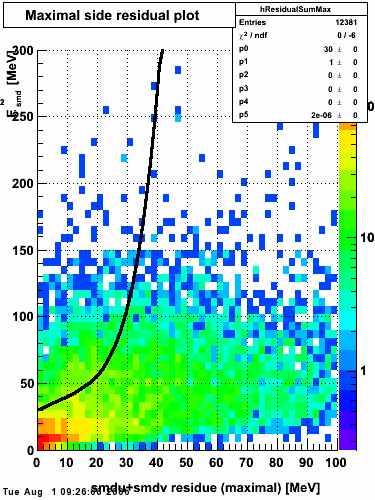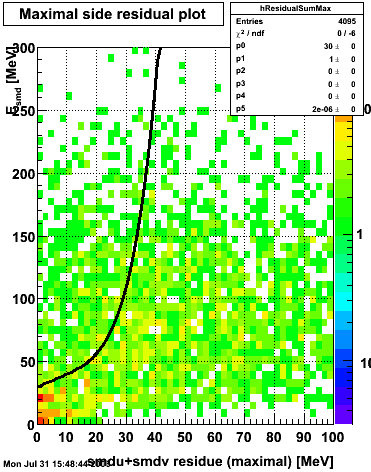Figure 1: Fitted peak integral vs. fit residual sum (U+V) from st_jpsi input stream (J/psi trigger only). Figure 2: Fitted peak integral vs. fit residual sum (U+V) from st_physics input stream (all triggers except express stream triggers).

## xy distribution of SMD hits

The separation between photons and pions was achieved by using Les cut in the above figures where photons reside above the curve and pions below. The data set used is the st_jpsi express stream.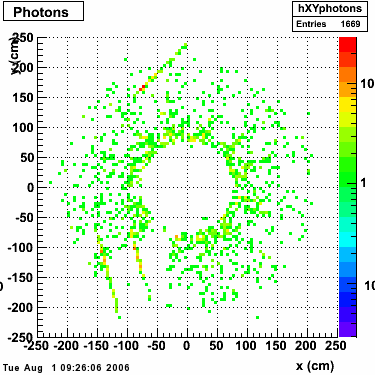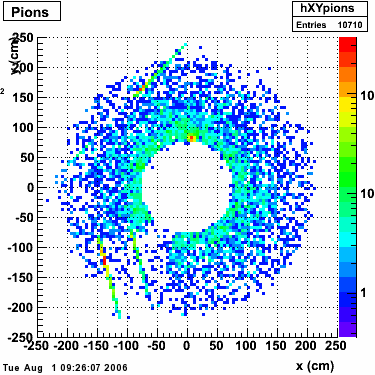## Single peak characteristics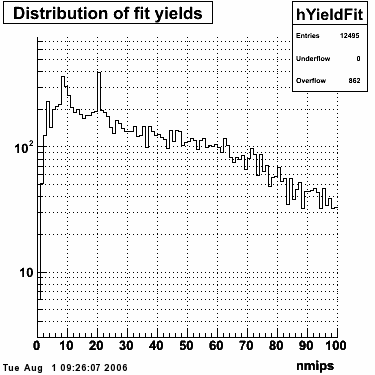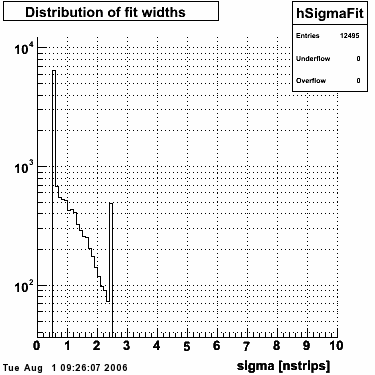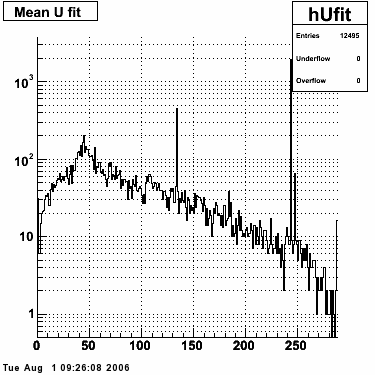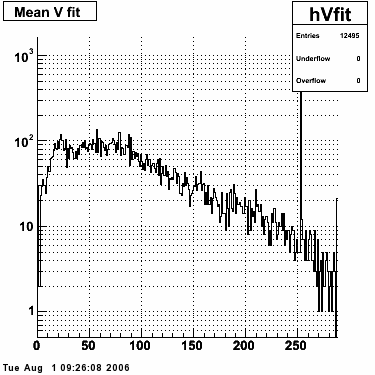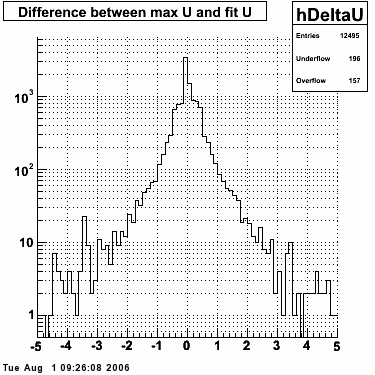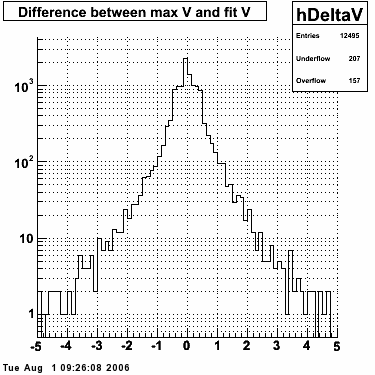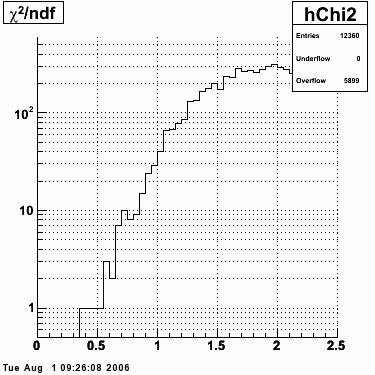## Fit function

The transverse profile of an electromagnetic shower in the SMD can be parametrized by the equation below in each SMD plane:f(x) is the energy in MeV as a function of SMD strip x. The algorithm performs a simultaneous fit in both the U and V plane. The maximal residual (data - fit) is then calculated. A single photon in the SMD should be well descibed by the equation above and therefore will have a smaller maximal residual. A neutral pion, which decays into two photons, should exhibit a larger maximal residual. Typically, the response would be a double peak, possibly a larger peak and a smaller peak corresponding to a softer photon.

## Single event SMD response

This directory contains images of single event SMD responses in both U and V plane. The file name convention is SMD_RUN_EVENT.png. The fit function for a single peak is the one described in the section above with 5 parameters:

• p0 = yield (P0), area under the peak in MeV
• p1 = mean (μ), center of peak in strips
• p2 = sigma of the first Gaussian (w1)
• p3 = fraction of the amplitude of the second Gaussian with respect to the first one (B), fixed to 0.2
• p4 = ratio of the width of the second Gaussian to the width of the first one (w2/w1), fixed to 3.5

macros